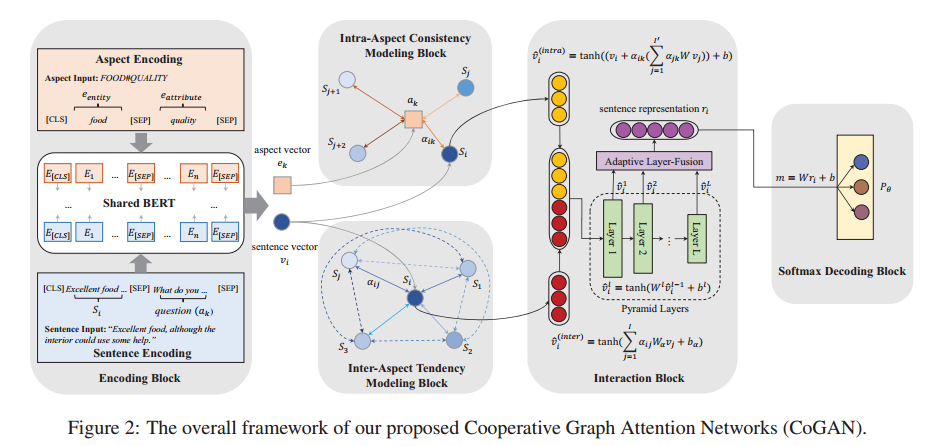Hope is a dangerous thing, but I have it.

# 【论文阅读】Aspect Sentiment Classification with Document-level Sentiment Preference Modeling (ACL 2020)

Aspect Sentiment Classification (ASC)

Price：positive
Food：negative

## Motivation1. contextual sentiment consistency (intra-aspect consistency)： 即对于一个文档，其中相同aspect的情感极性应该是一致的。
2. contextual sentiment tendency (inter-aspect tendency)： 即在同一个文档，各个aspect的情感极性应该是相似的。

## Method1. Encoding Block
用bert对sentence和aspect进行编码。
2. Intra-Aspect Consistency Modeling Block
• 建立GAN（二分图）：顶点是sentence+aspect，边是sentence和aspect的共现关系。
• 对于第k个aspect顶点 $a_k$，计算出每一个与它关联的sentence的权重。
$$\alpha_{ik} = \frac{exp(f(w^T[W_vv_i;W_ee_k]))}{\sum_{t=1}^{I'}{exp(f(w^T[W_vv_t;W_ee_k]))}}$$
• 然后根据权重进行加权求和，得到第k个aspect顶点 a_k的一个向量表示，再和原来的句子表示相加得到aspect-related sentence representation。
$$\hat{v}_i^{(intra)} = tanh((v_i+\alpha_{ik}(\sum_{j=1}^{I'}{\alpha_{jk}Wv_j}))+b)$$
1. Inter-Aspect Tendency Modeling Block
• 建立GAN（无向图）：顶点是sentence，边是sentence和sentence的共现关系（全连接）。
• 对于第i个sentence顶点 $s_i$，计算出每一个与它关联的sentence $s_j$的权重。
$$\alpha_{ij} = \frac{exp(f(w^T[W_1v_i;W_2v_j]))}{\sum_{t=1}^{I'}{exp(f(w^T[W_1v_i;W_2v_t]))}}$$
• 然后根据权重进行加权求和，得到i个sentence顶点 s_i的一个向量表示。
$$\hat{v}_i^{(inter)} = tanh(\sum_{j=1}^{I}{\alpha_{ij}W_{\alpha}v_j}+b_{\alpha})$$
1. Interaction Block
• Pyramid Layers：由于级联并不是一种很好的特征融合方法，所以这里采用了金字塔式的特征融合方法得到表示，就是将下一层的维度降到上一层的一半。